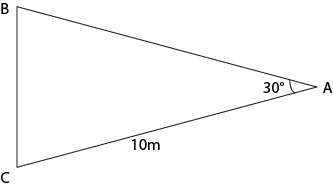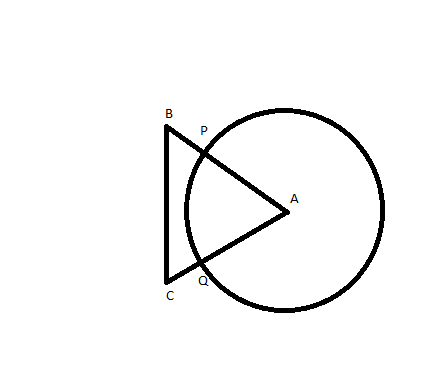### CAT 1998 Question Paper Question 54

Instructions

Answer the questions based on the following information. A cow is tethered at point A by a rope. Neither the rope nor the cow is allowed to enter ΔABC.
∠BAC = 30°
I(AB) = I(AC) = 10 mQuestion 54

# What is the area that can be grazed by the cow if the length of the rope is 8 m?

SolutionArea grazed by cow will be = (circle passing through P and Q) - (Area of circle inside the triangle)
= $$\pi r^2 - \frac{\pi r^2}{12}$$ = $$\frac{176\pi}{3}$$

• All Quant CAT Formulas and shortcuts PDF
• 30+ CAT previous papers with solutions PDF

##### Mayank Shekhar

2 years, 5 months ago

Explain it briefly so that anyone can understand it easily..

##### Himanshu jacker

3 years, 3 months ago

Bad solution I am not satisfying

OR